#### You may also likeMake a set of numbers that use all the digits from 1 to 9, once and once only. Add them up. The result is divisible by 9. Add each of the digits in the new number. What is their sum? Now try some other possibilities for yourself!### N000ughty Thoughts

How many noughts are at the end of these giant numbers?### DOTS Division

Take any pair of two digit numbers x=ab and y=cd where, without loss of generality, ab > cd . Form two 4 digit numbers r=abcd and s=cdab and calculate: {r^2 - s^2} /{x^2 - y^2}.

# Robotic Rotations

##### Age 11 to 16Challenge Level

Ashlynn from ISF Academy in Hong Kong created all of the patterns: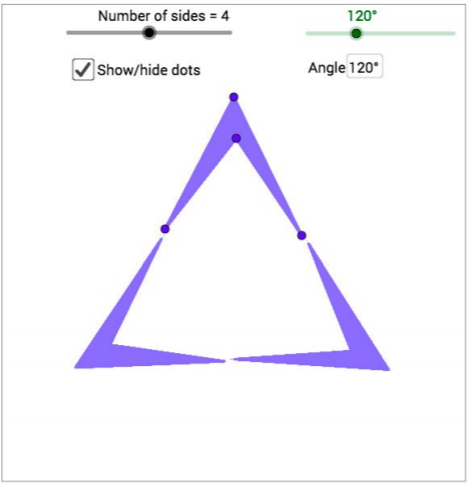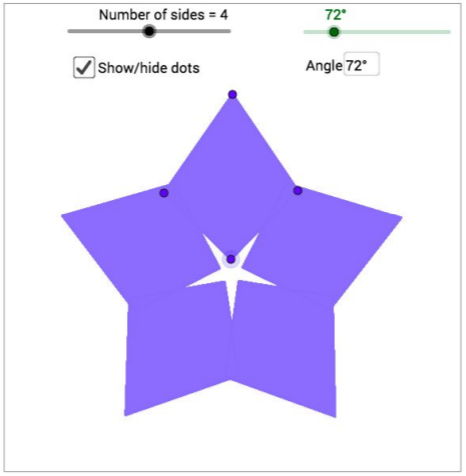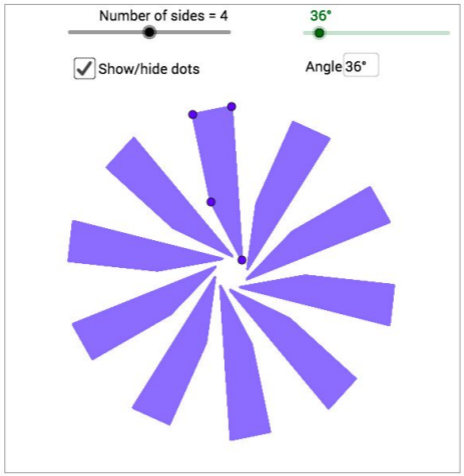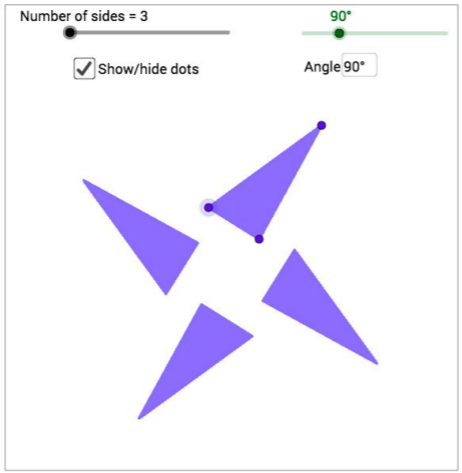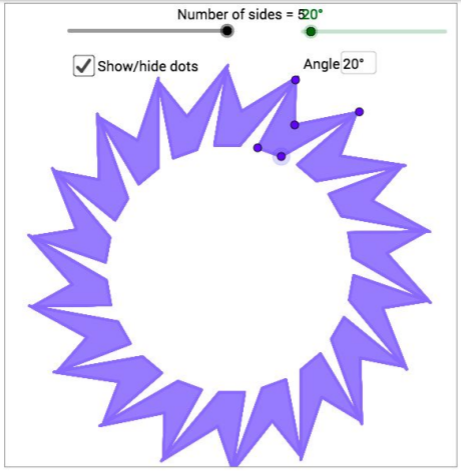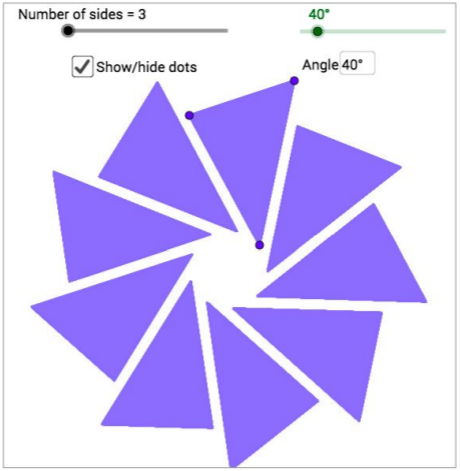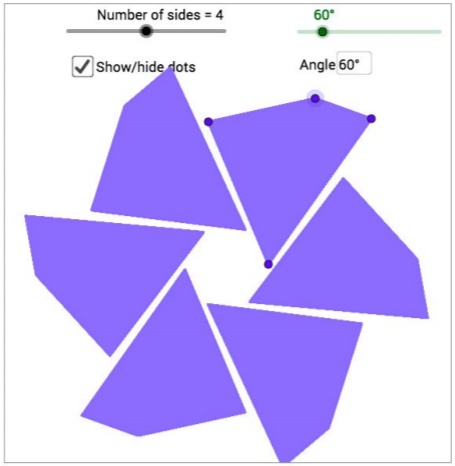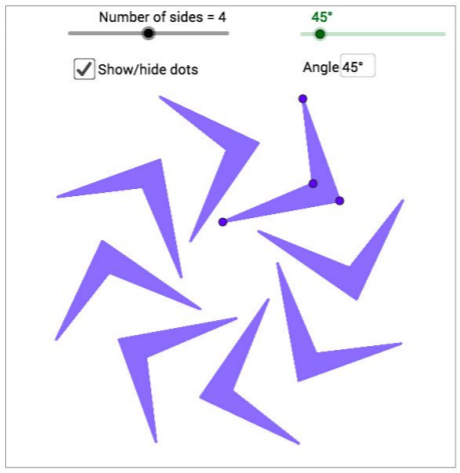For this picture, Ashylnn described which angles could have been used:
The smallest angle is 72$^\circ$
Multiples of 72$^\circ$ can also generate the same patternCan the other patterns be made using more than one angle? Ashlynn said:
Yes. In this picture, a rotation angle of 20$^\circ$ generates 18 shapes.
Other angles that are multiples of 20 (but not multiples of 40, 60) can generate the same pattern. These are 100$^\circ$, 140$^\circ$, 220$^\circ$, 260$^\circ$

Notice that this works because 18$\times$20$^\circ$ = 360$^\circ$, which is a full circle. So after 18 rotations of 20$^\circ$ (so 18 shapes), we get back to where we started from.

To make fewer copies of the shape, we need an angle with a multiple of 360 earlier in its times table. For example, above, 5$\times$72$^\circ$=360$^\circ$, so 72$^\circ$ only generates 5 copies of the shape.

5$\times$144 = 720 = 2$\times$360, so again after 5 copies rotating through 144$^\circ$, you get back to where you started. That is why multiples of 72$^\circ$ work.

However, Ashlynn pointed out that some multiples of 20$^\circ$ generate 18 copies of the shape, but those which are multiples of 40$^\circ$ do not.
This is because, although 18$\times$40$^\circ$ = 720$^\circ$ which is 2 full circles, 9$\times$40$^\circ$ = 360$^\circ$. So after just 9 copies, the robot gets back to where it started from and begins repeating the same shapes on top of each other.